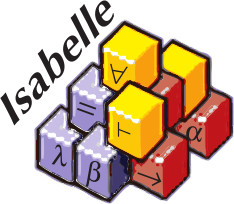# Common Errors

1. ```exception TYPE raised (line XXX of "envir.ML"): expand_atom: ill-typed replacement ```

This error can occur if you bind and use a pattern in a lemma statement, e.g.

``````lemma assumes "P foo" (is "P ?bar") shows "Q ?bar"
``````

This is due to the fact that parsing and type inference for the whole lemma statement is done at once.

Fix: Sufficiently verbose type annotations might help, e.g.

``````lemma assumes "P (foo ::'a)" (is "P ?bar") shows "Q (?bar :: 'a)"
``````
2. ```Bad Name: "t :: T" ```

When typing a definition and using type annotations at the wrong position, as in:

``````definition "a :: bool" where "a ≡ False"
``````

Isabelle returns `Bad name: "a :: bool"`.

Fix: use type annotations in the `where` clause instead:

``````definition "a" where "a :: bool ≡ False"
``````
3. ```Not an equation | Not a meta-equality (≡) ```

Most commonly, these errors occur because Isabelle's equality `=` binds relatively strongly. For example

``````definition d where "d b = b ∧ False"

fun f where "f b = b ∧ False"
``````

lead both to above error. Isabelle parses the term `t b = b ∧ False` as `(t b = b) ∧ False` and not `t b = (b ∧ False)`.

Fix: Explicitly set the parentheses, e.g. `t b = (b ∧ False)`